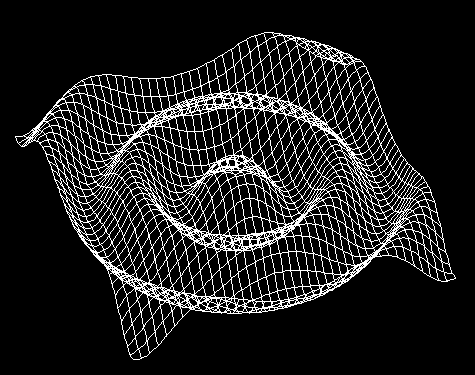Sine Wave Surface

This animation is a 3D surface plot of the function z = sin(sqrt(x2+y2)) or the height of the surface is determined from the sine of the distance from the z axis. To animate the wave I vary the phase of the sine function from 0 to 2pi.

Jamie Marconi